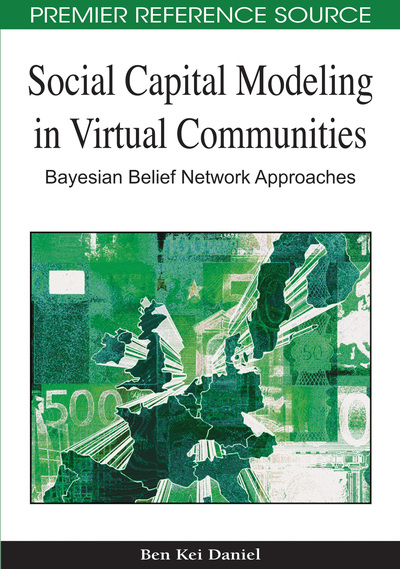# Model Validation

Ben Kei Daniel
DOI: 10.4018/978-1-60566-663-1.ch014
OnDemand:
(Individual Chapters)
Available
\$29.50
No Current Special Offers

## Abstract

Though computational models take a lot of effort to build, a model is generally not useful unless it can help people to understand the world being modelled, or the problem the model is intended to solve. A useful model allows people to make useful predictions about how the world will behave now and possibly tomorrow. Validation is the last step required in developing a useful Bayesian model. The goal of validation is to gain confidence in a model and to demonstrate and prove that a model produces reliable results that are closely related to the problems or issues in which the model is intended to address. The goal of the Chapter is to provide the reader with a basic understanding of the validation process and to share with them key lessons learned from the model of social capital presented in the book. While sensitivity analysis is intended to ensure that a Bayesian model is theoretically consistent with goals and assumptions of the modeller (how the modeller views the world) or the accuracy of sources of data used for building the model, the goal of validation is to demonstrate the practical application of the model in real world settings. This Chapter presents the main steps involved in the process of validating a Bayesian model. It illustrates this process by using examples drawn from the Bayesian model of social capital.
Chapter Preview
Top

## Procedures For Validating Model

Bayesian models are intended to predict or compare future performance of a system. Model validation is concerned with ensuring the model built is an accurate representation of a real system in a real world and that outcomes of its predictions can be applied to solve set of problems or inform us about operation of a certain phenomenon.

Model validation is achieved through the calibration of the model, which essentially compares the model to actual system behaviour and using the discrepancies between the two, and the insights gained from the process to improve or disapprove the model. This process is repeated until model accuracy is judged to be acceptable. Further, the validation process is contingent upon the model developer and people knowledgeable of how the real system works. Model validation is performed through evaluation of accuracy of the model with respect to experimental data.

There are many approaches for validating Bayesian model but it is beyond the scope of this book to elaborate on each of these approaches. One could certainly generalize that unlike mathematical models, where there are well-established procedures and to some extent concrete guidelines for validating a mathematical model, the guidelines for validating Bayesian models of social systems are inadequate and to some extent difficult to carry out.

There were two different possible ways to validate the model of social capital, one was to use an expert driven validation technique, intended to validate exploratory process involved in the model building. The second technique was an empirical driven model validation, the result of which is the highlights of some of the components of social capital discussed. The expert driven model validation involved collecting sufficient empirical data to corroborate against predictions of a model, which were based on the experts’ knowledge and reflected in the initial development of the model. The validation of empirical part compared the model input-output predictions to corresponding input-output transformations for a real system and it was achieved through previous work referred to throughout the book. Figure 1 presents a general process of model validation; this can be applied to almost any Bayesian model.

Top

## Validating A Bayesian Model

A Bayesian model is considered valid when a set of experimental conditions is performed against it to determine the model’s accuracy within its intended purpose. This usually requires that the model’s output variables of interest are identified and that their required amount of accuracy be specified. The amount of accuracy required is normally specified prior to starting the development of the model or at a very early in the model development process. If the variables of interest are random variables, then properties and functions of the random variables such as means and variances are usually used to determine the model validity. In practice when dealing Bayesian models of social systems, several iterations of validations are required to obtain a satisfactorily valid model.

## Complete Chapter List

Search this Book:
Reset